Next: Rational filters Up: DAMPED OSCILLATION Previous: Polynomial division

## Spectrum of a pole

Now that we have seen the single-pole filter and the pole-pair filter in both the time domain and the frequency domain, let us find their analytical expressions. Taking the pole to be, we have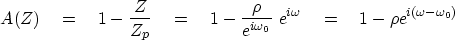(35)
The complex conjugate is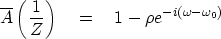(36)
The spectrum of a pole filter is the inverse of(37)
With the definition of a small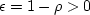, inverting gives(38)
Specializing to frequencies close to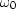,where the denominator is small and the function is large, gives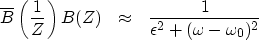(39)

This is called a narrow-band filter" because in the Fourier domain

the function is large only in a narrow band of frequencies. Settingto half its peak value of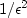,we find a half-bandwidth of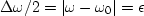.The damping time constantof the damped sinusoid bt is shown in the exercises following this section to be.

Naturally we want a real-time function, so we multiply the filter 1/(1-Z/Zp) times.The resulting time function is real because conjugate poles are like the conjugate roots. The spectrum of the conjugate factoris like (38), except thatis replaced by.Multiplying the response (38) by itself withyields the symmetric function ofdisplayed on the right in Figure 9.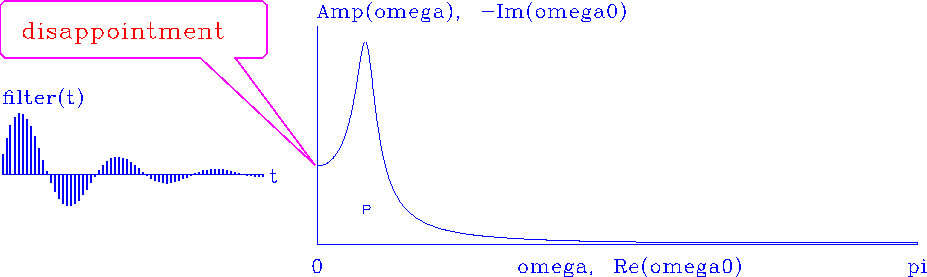disappoint
Figure 9
A pole near the real axis gives a damped sinusoid in time on the left. On the right isforreal.

You might be disappointed if you intend to apply the filter of Figure 9 as a narrow-band filter. Notice that the passband is asymmetric and that it passes the zero frequency. Equation (38) is symmetric about,but taking the product with its image abouthas spoiled the symmetry. Should we be concerned about this edge effect''? The answer is yes, whenever we handle real data. For real data,is usually small enough. Recall that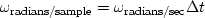.Consider a pole at a particular:decreasingpushestowards zero, which is where a pole and its mate at negative frequency create the asymmetrical response shown in Figure 9.

So in practice we might like to add a zero at zero frequency and at the Nyquist frequency, i.e., (1-Z)(1+Z), as shown in Figure 10.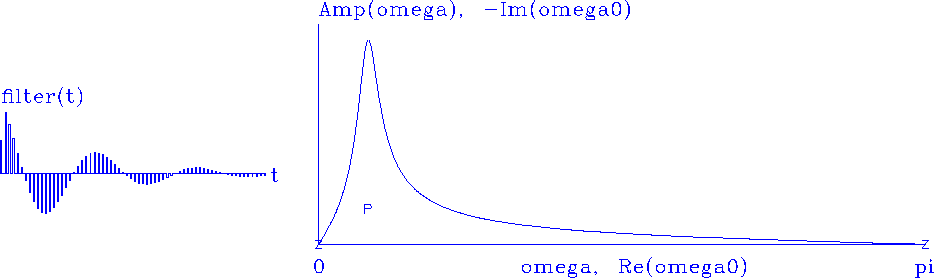symdsin
Figure 10
Poles at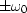; a root atand another root at.

Compare Figure 10 and 9. If the time functions were interchanged, could you tell the difference between the figures? There are two ways to distinguish them. The most obvious is that the zero-frequency component is made evident in the time domain by the sum of the filter coefficients (theoretically, F(Z=1)). A more subtle clue is that the first half-cycle of the wave in Figure 10 is shorter than in Figure 9; hence, it contains extra high frequency energy, which we can see in the spectrum.

## EXERCISES:

1. Sketch the function in equation (38) over the range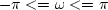, taking care to distinguish it from Figure 9.
2. Figure 9 shows a bump aroundthat does not look symmetric because it is the product of equation (38) with a frequency-reversed copy. Consider the sum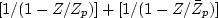.Is the time filter real? Where are its poles and zeros? How will its amplitude as a function of frequency compare with the amplitude of Figure 9? Will the bump look more symmetric?Next: Rational filters Up: DAMPED OSCILLATION Previous: Polynomial division
Stanford Exploration Project
10/21/1998